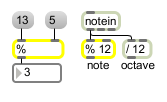%

Divide two numbers, output the remainder

Description

% takes two numbers, divides one by the other and outputs the remainder of the division.

Arguments

Name Type Opt Description
initial-value int opt Sets an initial value for the divisor. If there is no argument, the divisor is set to 1 initially.

Messages

 bang In left inlet: Performs the operation with the numbers currently stored. int input [int] In left inlet: The number is divided by the number in the right inlet, and the remainder is sent out the outlet. (inlet1) divisor [int] In right inlet: The number is stored as the divisor (the number to be divided into the number in the left inlet) for calculating the remainder. float input [float] Converted to int. set set-input [int] Sets the number to be divided without causing output (bang will output it). list number-divided and divisor [list] In left inlet: The first number is divided by the second number, and the remainder is sent out the outlet.

Output

int: When the two numbers in the inlets are divided, the remainder is sent out the outlet. % is called the modulo operator.

ExamplesFind the remainder of a division